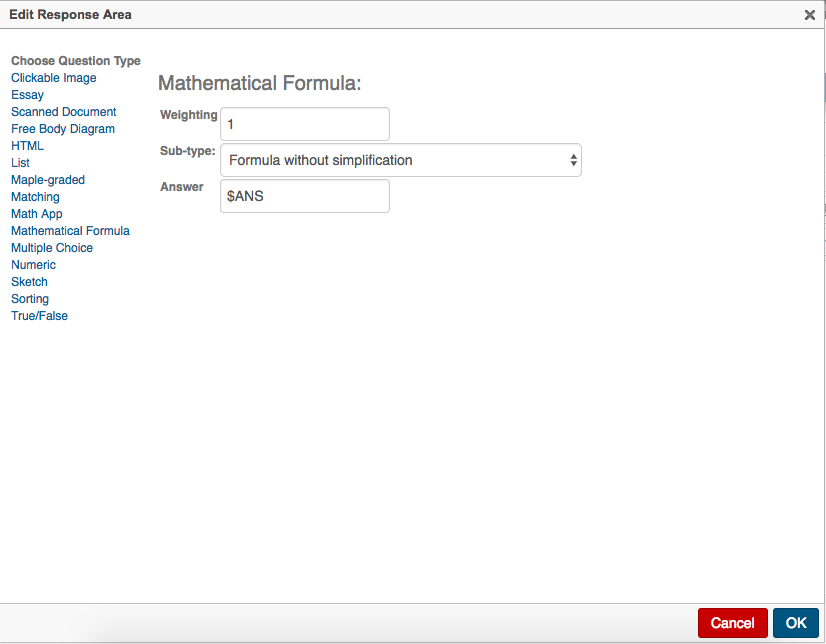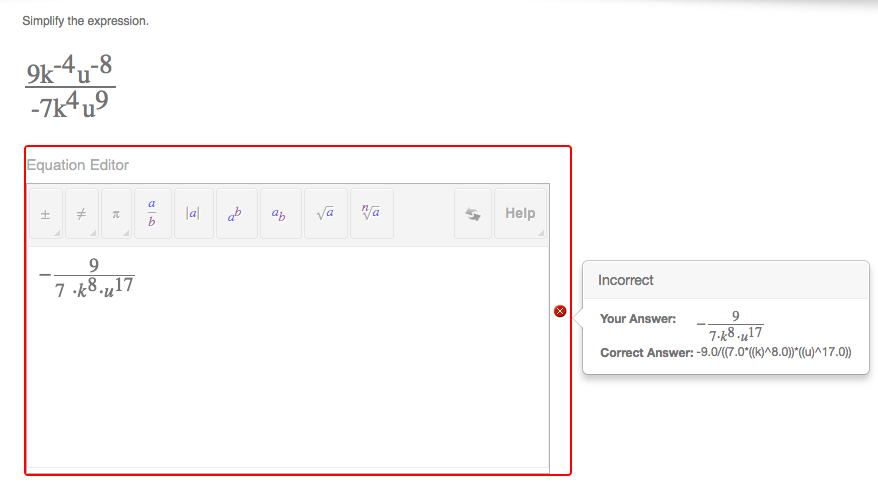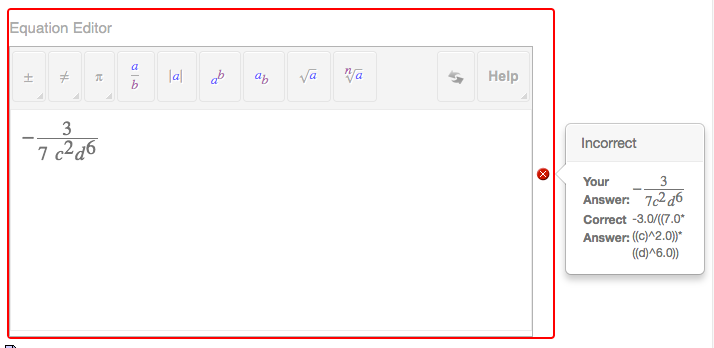## 40 Reputation

5 years, 130 days

## @kat05 I am unsure of what you mean, spe...

@kat05 I am unsure of what you mean, specifically because the answer key in my above example WAS generated with randomized variables, then I went in with the preview function to test.

I have done this in NUMEROUS other problems...but it doesn't seem to assess correctly on this problem versus the others. Here's my response area:My code for setting the \$ANS variable for the scenario BELOW looks like this (I'm going to pull it out of the if / switch embedding to ensure the proper placement of variables):

\$ANS = \$C1a / ( \$C2a * \$V1^abs(\$Aa) * \$V2^abs(\$Ba) );

The thing is, this SEEMS to work fine. The "correct answer" section in question preview is the correct answer...but the question type will not evaluate my response as a correct response. I don't want to use the regular formula option since--obviously--it'd allow you to put in the question itself and be marked correct. Putting in multiplication doesn't seem to change anything, unfortunately.I'd be totally willing to swap this question type out for maple graded, though...can you walk me through how I'd write a maple graded response and specify no simplification, presuming the answer is the formula "\$ANS"?

## @kat05 when I write an equation as ...

@kat05 when I write an equation as my output, we come up with this issue...even though the answer I gave and the answer they have are the same...they're different...because of parentheses, I guess? =/.## Thanks for taking a look =)....

@kat05 I often use variables in the answers to such problems--but rarely ones as complex as this. As a warning, I'll wager there are like 15 specialty function calls that will do similar things to what I'm doing with a single call...my background is C++ from decades ago, so I'm sure my methods are inefficient.

As a note, all R variables are "randomizers." Since I want to compare before switches and you can't set a variable after its initial setting, it became necessary for me (though probably not necessary in general...unsure on that,) to declare 6 variables that are otherwise unused in the problem.

The problem is simply

Simplify the expression.

\$C1\$V1\$A1\$V2\$B1
\$C2\$V1\$A2\$V2\$B2

Here's all the code I use to write the problem.

#CODE TO SELECT TWO VARIABLES IN ALPHABETICAL ORDER WITHOUT TROUBLE LETTERS
\$R = rint( 21 );
\$R0 = rint( 22 );

\$R1 = if ( lt( \$R, \$R0 ), \$R, \$R0 );
\$R2 = if ( lt( \$R, \$R0 ), \$R0, \$R + 1 );

\$V1 = switch( \$R1,a,b,c,d,f,g,h,j,k,m,n,p,q,r,s,t,u,v,w,x,y,z );
\$V2 = switch( \$R2,a,b,c,d,f,g,h,j,k,m,n,p,q,r,s,t,u,v,w,x,y,z );

#Either the first, second, or both exponents are negative for each variable.
\$R3 = rint( 3 );
\$R4 = rint( 3 );

\$A1 = switch(  \$R3, -1*( rint( 9 ) + 1 ), rint( 8 ) + 2, -1*( rint( 9 ) + 1 ) );
\$A2 = switch(  \$R3, rint( 8 ) + 2, -1*( rint( 9 ) + 1 ), -1*( rint( 9 ) + 1 ) );

\$B1 = switch(  \$R4, -1*( rint( 9 ) + 1 ), rint( 8 ) + 2, -1*( rint( 9 ) + 1 ) );
\$B2 = switch(  \$R4, rint( 8 ) + 2, -1*( rint( 9 ) + 1 ), -1*( rint( 9 ) + 1 ) );

#Create our constants and their print variants.
\$C1 = ( rint( 9 ) + 1 ) * -1^( rint( 3 ) );
\$C2 = ( rint( 9 ) + 1 ) * -1^( rint( 3 ) );

\$C1p = if( \$C1 - 1, if( \$C1 + 1, \$C1, "-" ), "" );
\$C2p = if( \$C2 - 1, if( \$C2 + 1, \$C2, "-" ), "" );

#Here's where the code would go to write the answer, but my code isn't working properly!
#Or at least isn't working with the answer type =/.
#Calculate the numerator

\$ANSn = if( lt( \$Aa, 1 ),
if( lt( \$Ba, 1 ), \$C1a,
if( gt( abs(\$C1a), 1), -\$C1a*\$V2^\$Ba,
if( lt( \$C1a, 0 ), -\$V2^\$Ba, \$V2^\$Ba ) ) ),
if( lt( \$Ba, 1 ),
if( gt( abs(\$C1a), 1), \$C1a*\$V1^\$Aa,
if( lt( \$C1a, 0 ), -\$V1^\$Aa, \$V1^\$Aa ) ),
if( gt( abs(\$C1a), 1), \$C1a* \$V1^\$Aa * \$V2^\$Ba,
if( lt( \$C1a, 0 ), -\$V1^\$Aa * \$V2^\$Ba, \$V1^\$Aa * \$V2^\$Ba ) ) ) );

#Divide that by our denominator.

\$ANS = if( lt( \$Aa, 0 ),
if (lt (\$Ba, 0 ),
\$ANSn / ( \$C2a*( \$V1^(abs( \$Aa ) ) ) * ( \$V2^(abs( \$Ba ) ) ) ),
\$ANSn / ( \$C2a*( \$V1^(abs( \$Aa ) ) ) ) ),
if (lt (\$Ba, 0 ),
\$ANSn / ( \$C2a* \$V2^(abs( \$Ba ) ) ),
if( gt( \$C2a, 1 ), \$ANSn / \$C2a, \$ANSn ) ) );

## @BorisP I've gotten it working by settin...

@BorisP I've gotten it working by setting up my answer before parsing as a fraction or as a whole number before shipping ot the answer section. I really appreciate your help on this one! It boggled me for quite a while =).

## @BorisP Neat! Worked like a charm!Im loo...

@BorisP Neat! Worked like a charm!

Im looking up more information on numfmt...looks like that's got a bunch of possible uses. Thanks for the help!

## Parsing issue with fraction given...

@BorisP When we get a whole number answer, the code given yields an error.

I can reduce the fraction myself within the algorithm section, but the issue is when the fraction yields a whole number--e.g. 3--the student would be required to write 3/1.

## @Doug Meade The if function evaluates ba...

@Doug Meade The if function evaluates based upon is the value zero, returning one if the value is zero, or the original value if it's not. Your solution is most definitely cleaner, so I'll be running that instead =).

## @BorisP Thanks so much for your help! I'...

@BorisP Thanks so much for your help! I'll give it a go now!

## @Doug Meade will give it a look! I've be...

@Doug Meade will give it a look! I've been avoiding the function editor for these sets of problems because I'm making a series of questions that could go very low for student ages, and the buttons might be pretty intimidating. I'll check it out =).

## Thanks @BorisP! ...

Thanks @BorisP

 Page 1 of 1
﻿